#### Linear sensing models

Developed mainly in control theory literature, linear sensing models are some of the most common and important. For all of the sensors in this family, assume that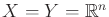(nonsingular linear transformations allow the sensor space to effectively have lower dimension, if desired). The simplest case in this family is the identity sensor, in which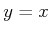. In this case, the state is immediately known. If this sensor is available at every stage, then the I-space collapses toby the I-map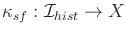.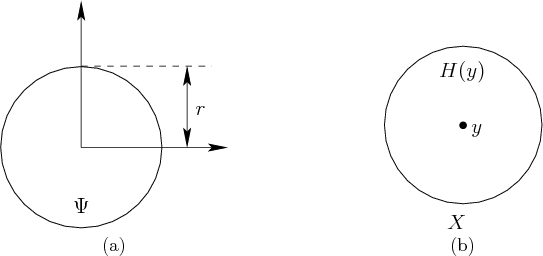Now nature sensing actions can be used to corrupt this perfect state observation to obtain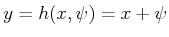. Suppose that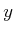is an estimate of, the current state, with error bounded by a constant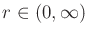. This can be modeled by assigning for every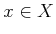,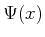as a closed ball of radius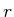, centered at the origin: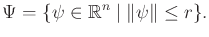(11.67)

Figure 11.11 illustrates the resulting nondeterministic sensing model. If the observationis received, then it is known that the true state lies within a ball inof radius, centered at. This ball is the preimage,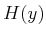, as defined in (11.11). To make the model probabilistic, a probability density function can be defined over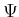. For example, it could be assumed that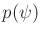is a uniform density (although this model is not very realistic in many applications because there is a boundary at which the probability mass discontinuously jumps to zero).

A more typical probabilistic sensing model can be made by letting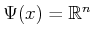and defining a probability density function over all of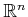. (Note that the nondeterministic version of this sensor is completely useless.) One of the easiest choices to work with is the multivariate Gaussian probability density function,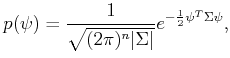(11.68)

in which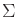is the covariance matrix (11.64),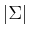is its determinant, and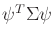is a quadratic form, which multiplies out to yield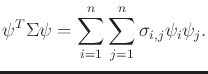(11.69)

If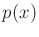is a Gaussian andis received, then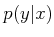must also be Gaussian under this model. This will become very important in Section 11.6.1.

The sensing models presented so far can be generalized by applying linear transformations. For example, let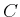denote a nonsingular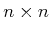matrix with real-valued entries. If the sensor mapping is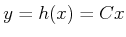, then the state can still be determined immediately because the mapping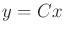is bijective; eachcontains a unique point of. A linear transformation can also be formed on the nature sensing action. Let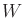denote anmatrix. The sensor mapping is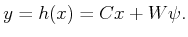(11.70)

In general,andmay even be singular, and a linear sensing model is still obtained. Suppose that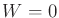. Ifis singular, however, it is impossible to infer the state directly from a single sensor observation. This generally corresponds to a projection from an-dimensional state space to a subset of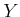whose dimension is the rank of. For example, if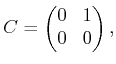(11.71)

thenyields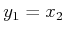and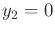. Only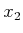of each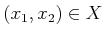can be observed becausehas rank. Thus, for some special cases, singular matrices can measure some state variables while leaving others invisible. For a general singular matrix, the interpretation is thatis projected into some-dimensional subspace by the sensor, in whichis the rank of. Ifis singular, this means that the effect of nature is limited. The degrees of freedom with which nature can distort the sensor observations is the rank of. These concepts motivate the next set of sensor models.

Steven M LaValle 2020-08-14